CBSE Class 12 Sample Paper for 2019 Boards

Class 12
Solutions of Sample Papers and Past Year Papers - for Class 12 Boards

Question 25

A cuboidal shaped godown with square base is to be constructed. Three times as much cost per square meter is incurred for constructing the roof as compared to the walls. Find the dimensions of the godown if it is to enclose a given volume and minimize the cost of constructing the roof and the walls.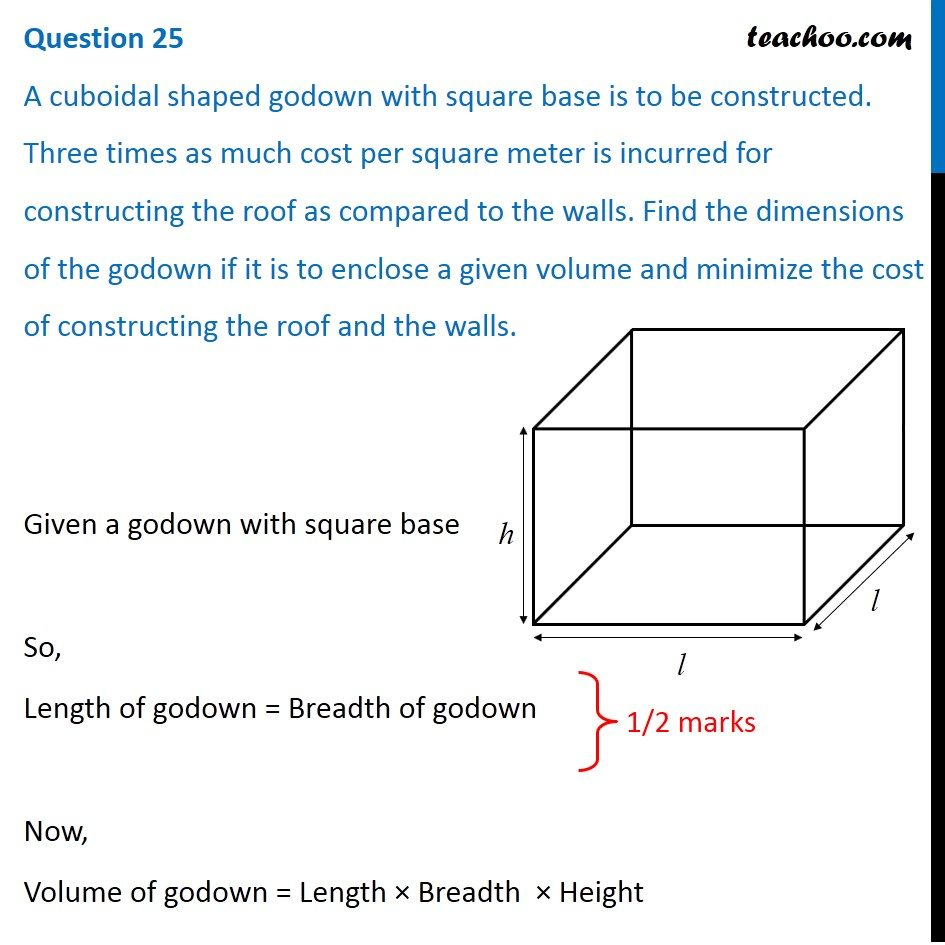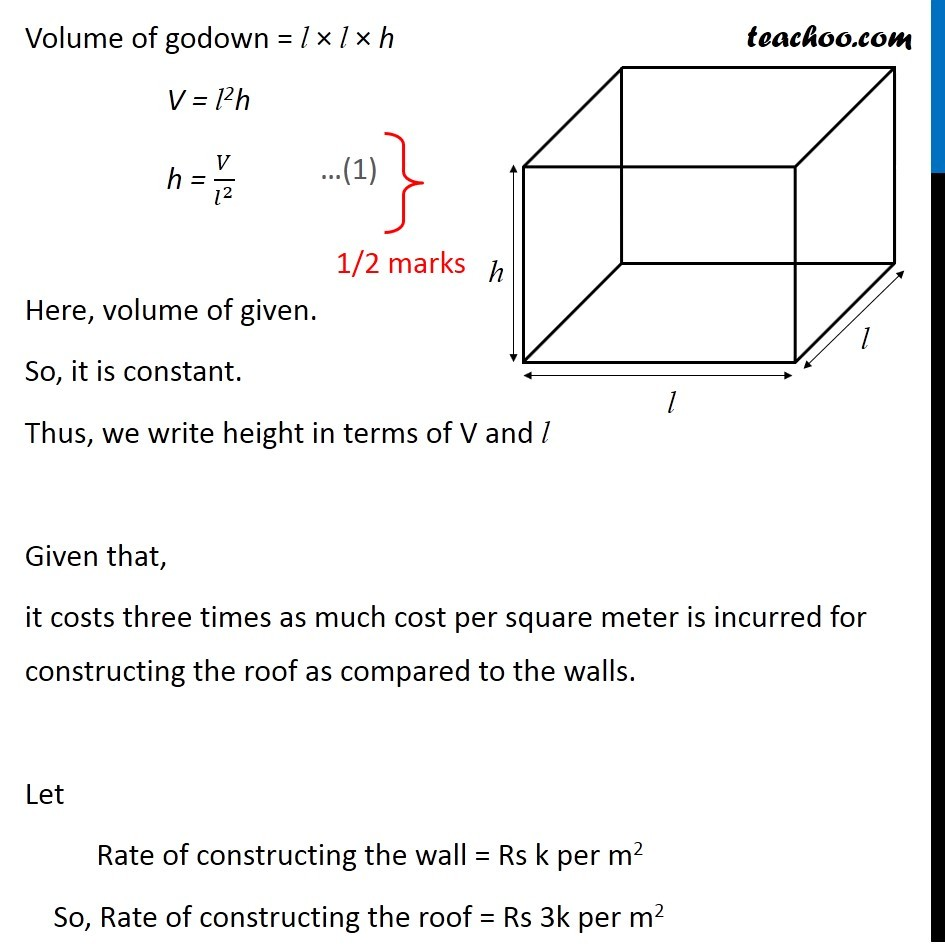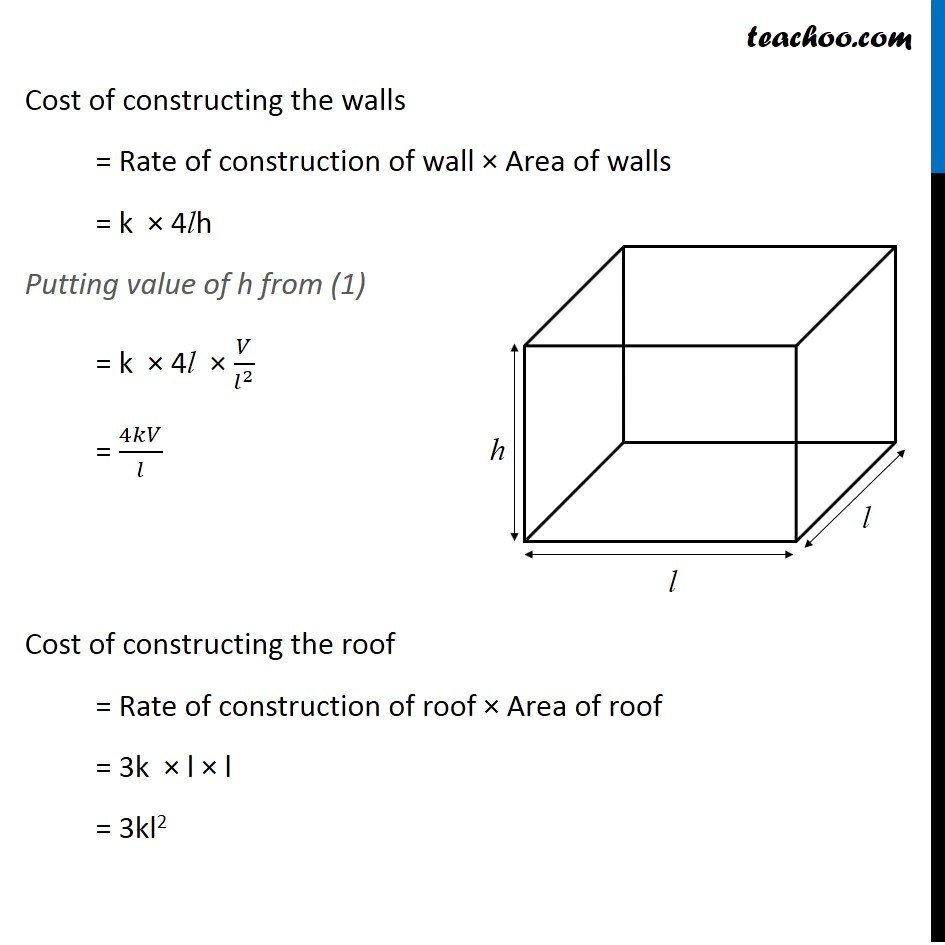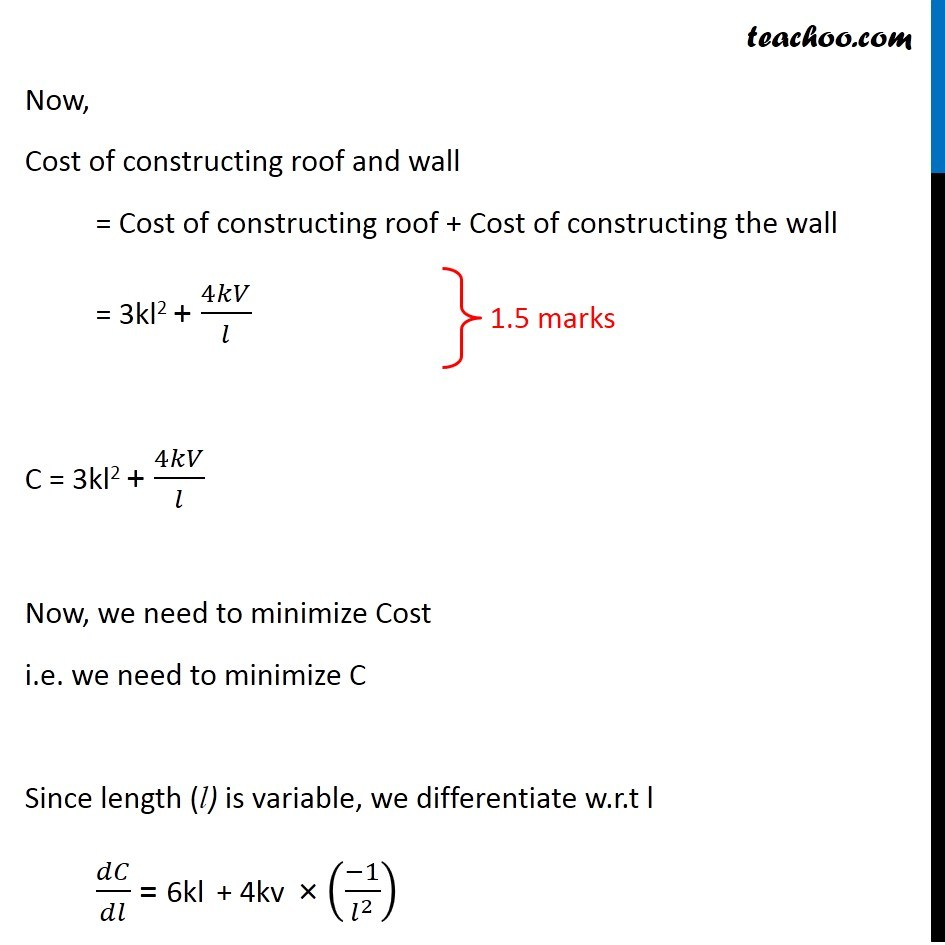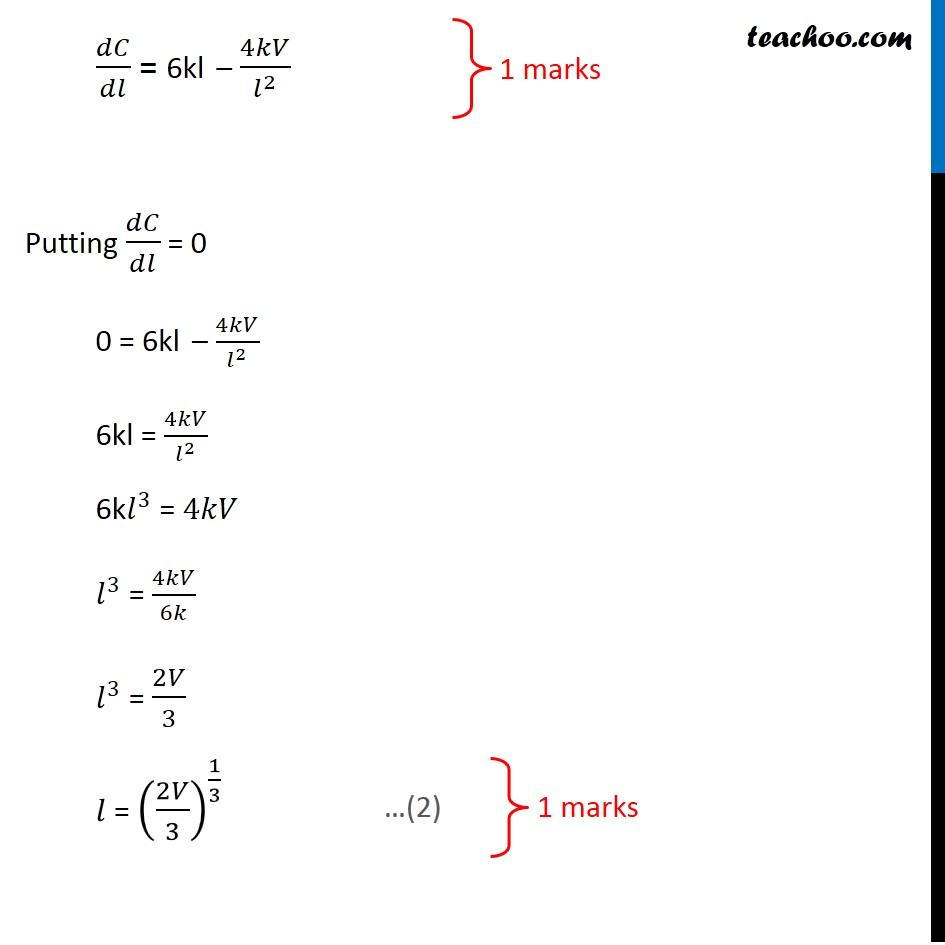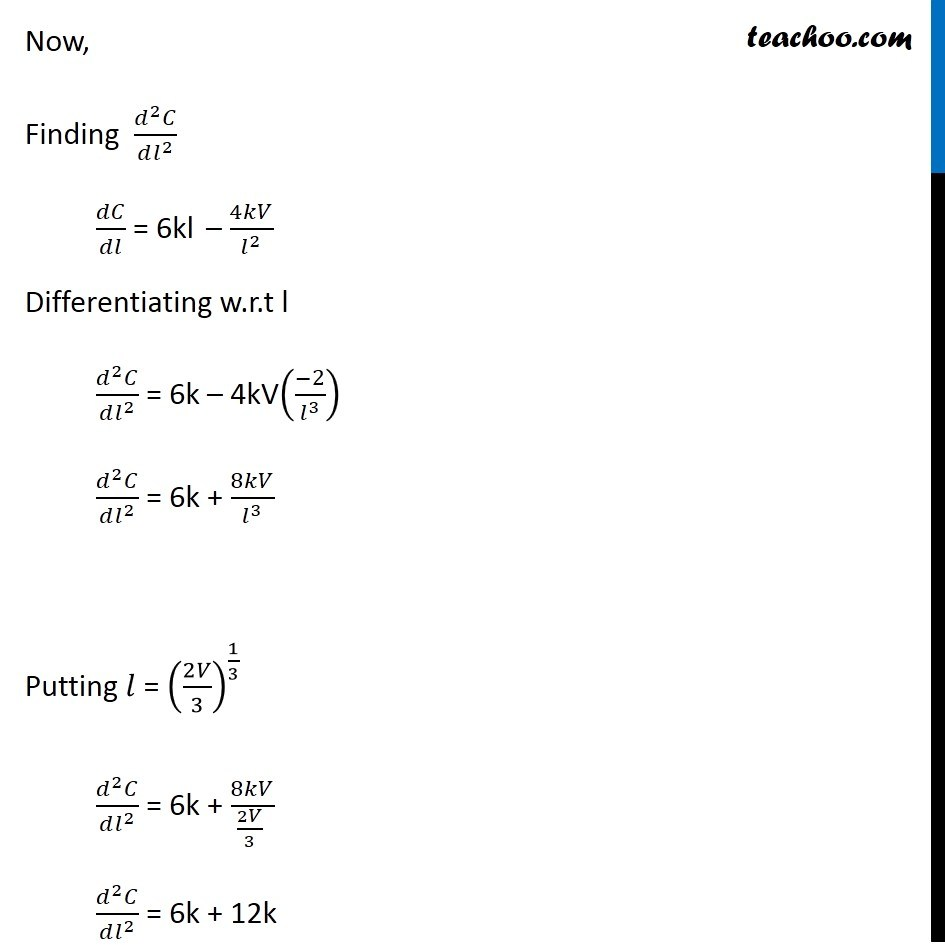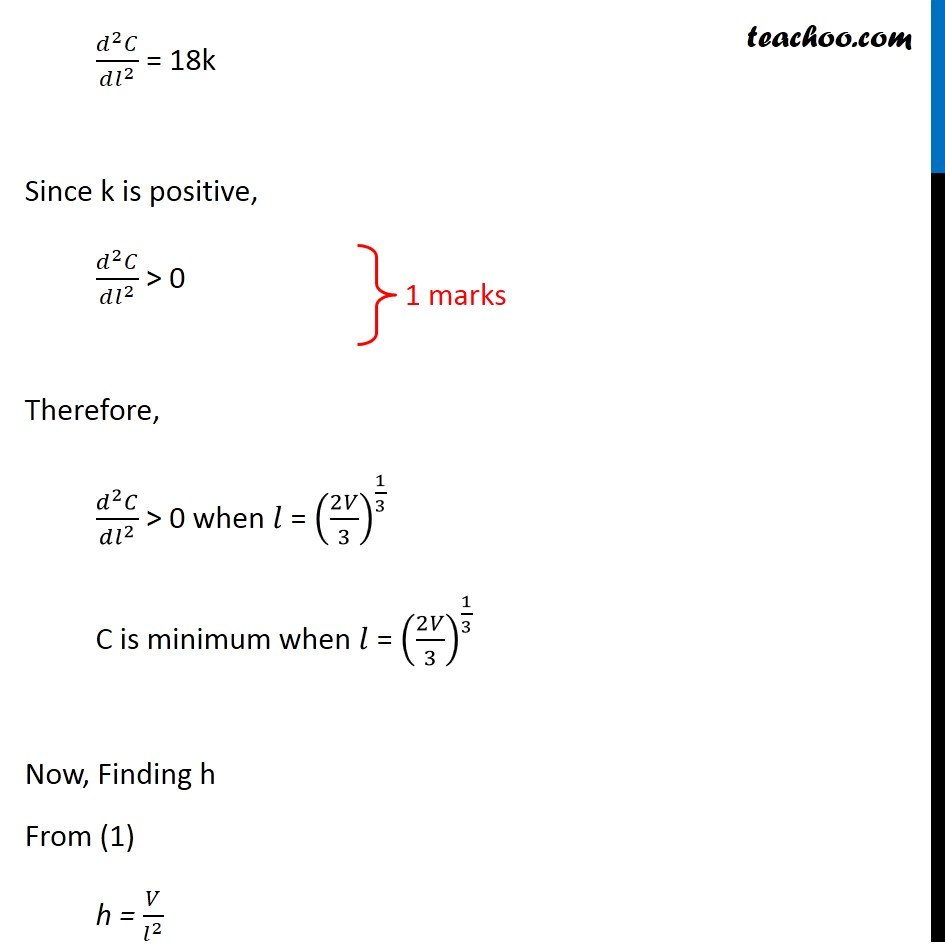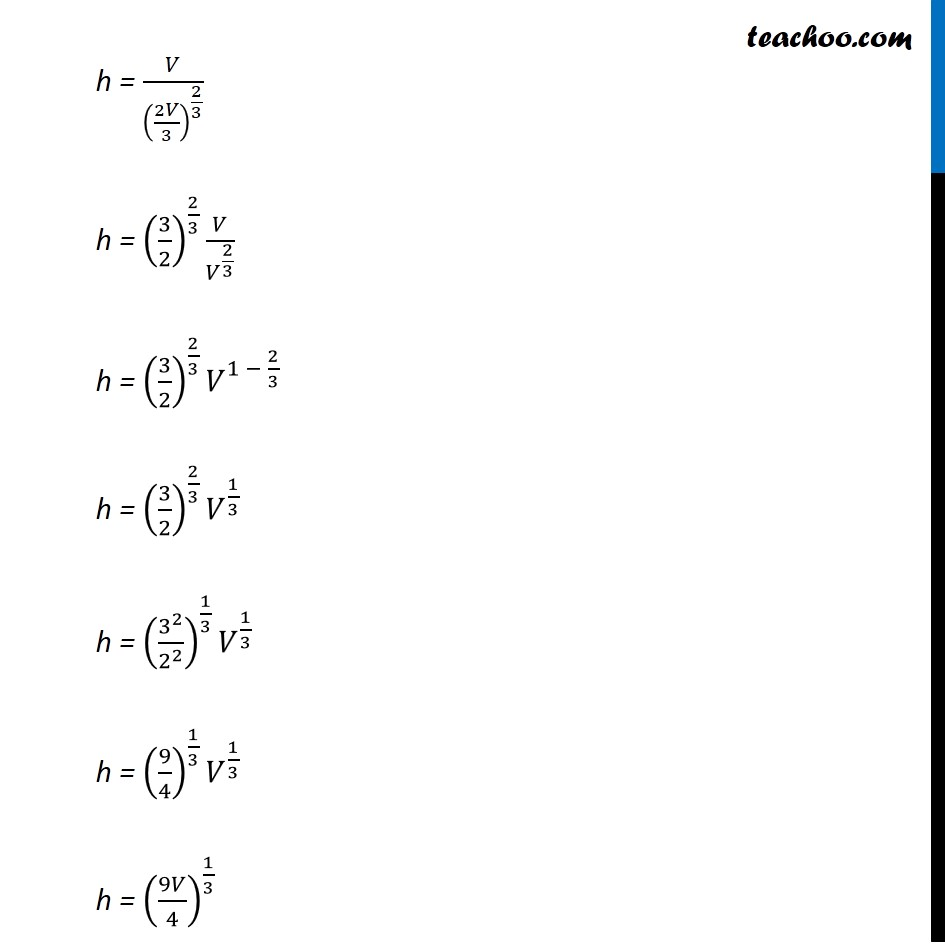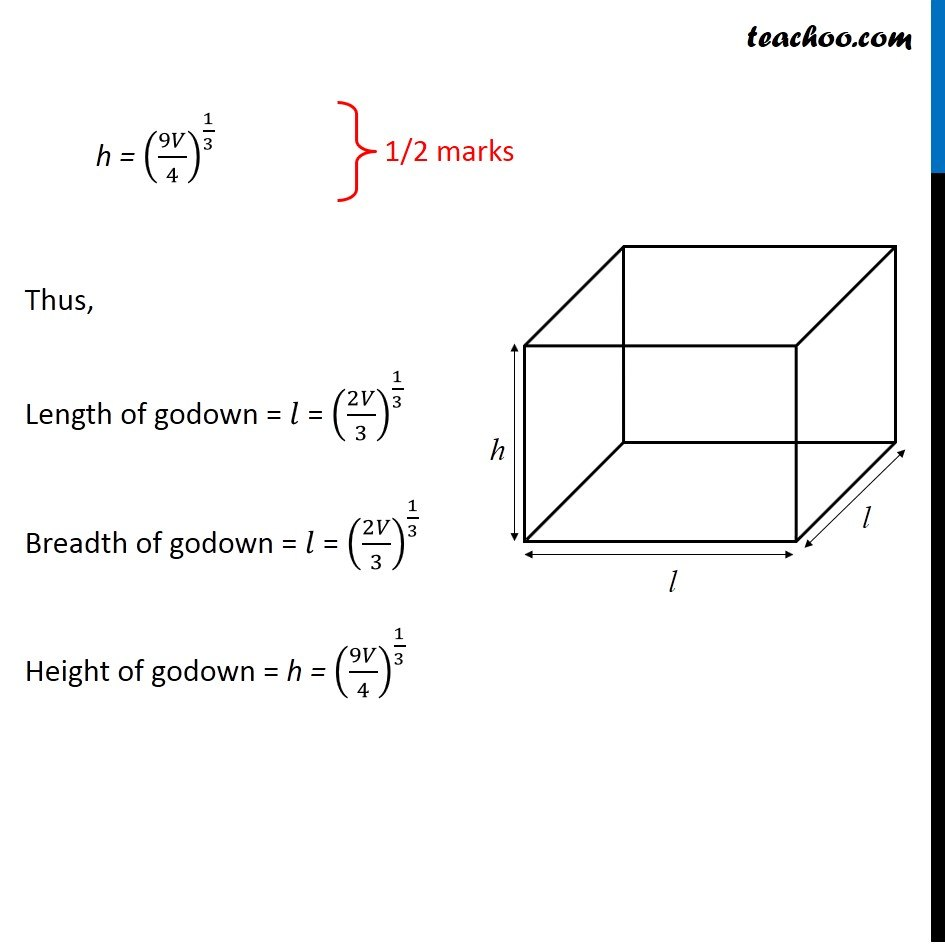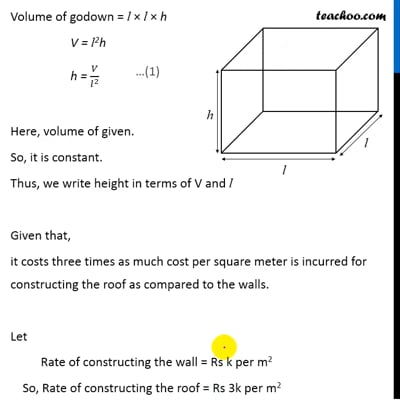This video is only available for Teachoo black users

Learn in your speed, with individual attention - Teachoo Maths 1-on-1 Class

### Transcript

Question 25 A cuboidal shaped godown with square base is to be constructed. Three times as much cost per square meter is incurred for constructing the roof as compared to the walls. Find the dimensions of the godown if it is to enclose a given volume and minimize the cost of constructing the roof and the walls. Given a godown with square base So, Length of godown = Breadth of godown Now, Volume of godown = Length × Breadth × Height Volume of godown = l × l × h V = l2h h = 𝑉/𝑙^2 Here, volume of given. So, it is constant. Thus, we write height in terms of V and l Given that, it costs three times as much cost per square meter is incurred for constructing the roof as compared to the walls. Let Rate of constructing the wall = Rs k per m2 So, Rate of constructing the roof = Rs 3k per m2 Cost of constructing the walls = Rate of construction of wall × Area of walls = k × 4lh Putting value of h from (1) = k × 4l × 𝑉/𝑙^2 = 4𝑘𝑉/𝑙 Cost of constructing the roof = Rate of construction of roof × Area of roof = 3k × l × l = 3kl2 Now, Cost of constructing roof and wall = Cost of constructing roof + Cost of constructing the wall = 3kl2 + 4𝑘𝑉/𝑙 C = 3kl2 + 4𝑘𝑉/𝑙 Now, we need to minimize Cost i.e. we need to minimize C Since length (l) is variable, we differentiate w.r.t l 𝑑𝐶/𝑑𝑙 = 6kl + 4kv × ((−1)/𝑙^2 ) 𝑑𝐶/𝑑𝑙 = 6kl – 4𝑘𝑉/𝑙^2 Putting 𝑑𝐶/𝑑𝑙 = 0 0 = 6kl – 4𝑘𝑉/𝑙^2 6kl = 4𝑘𝑉/𝑙^2 6k𝑙^3 = 4𝑘𝑉 𝑙^3 = 4𝑘𝑉/6𝑘 𝑙^3 = 2𝑉/3 𝑙 = (2𝑉/3)^(1/3) Now, Finding (𝑑^2 𝐶)/(𝑑𝑙^2 ) 𝑑𝐶/𝑑𝑙 = 6kl – 4𝑘𝑉/𝑙^2 Differentiating w.r.t l (𝑑^2 𝐶)/(𝑑𝑙^2 ) = 6k – 4kV((−2)/𝑙^3 ) (𝑑^2 𝐶)/(𝑑𝑙^2 ) = 6k + 8𝑘𝑉/𝑙^3 Putting 𝑙 = (2𝑉/3)^(1/3) (𝑑^2 𝐶)/(𝑑𝑙^2 ) = 6k + 8𝑘𝑉/(2𝑉/3 ) (𝑑^2 𝐶)/(𝑑𝑙^2 ) = 6k + 12k (𝑑^2 𝐶)/(𝑑𝑙^2 ) = 18k Since k is positive, (𝑑^2 𝐶)/(𝑑𝑙^2 ) > 0 Therefore, (𝑑^2 𝐶)/(𝑑𝑙^2 ) > 0 when 𝑙 = (2𝑉/3)^(1/3) C is minimum when 𝑙 = (2𝑉/3)^(1/3) Now, Finding h From (1) h = 𝑉/𝑙^2 h = 𝑉/(2𝑉/3)^(2/3) h = (3/2)^(2/3) 𝑉/𝑉^(2/3) h = (3/2)^(2/3) 𝑉^(1 − 2/3) h = (3/2)^(2/3) 𝑉^(1/3) h = (3^2/2^2 )^(1/3) 𝑉^(1/3) h = (9/4)^(1/3) 𝑉^(1/3) h = (9𝑉/4)^(1/3) h = (9𝑉/4)^(1/3) Thus, Length of godown = 𝑙 = (2𝑉/3)^(1/3) Breadth of godown = 𝑙 = (2𝑉/3)^(1/3) Height of godown = h = (9𝑉/4)^(1/3)